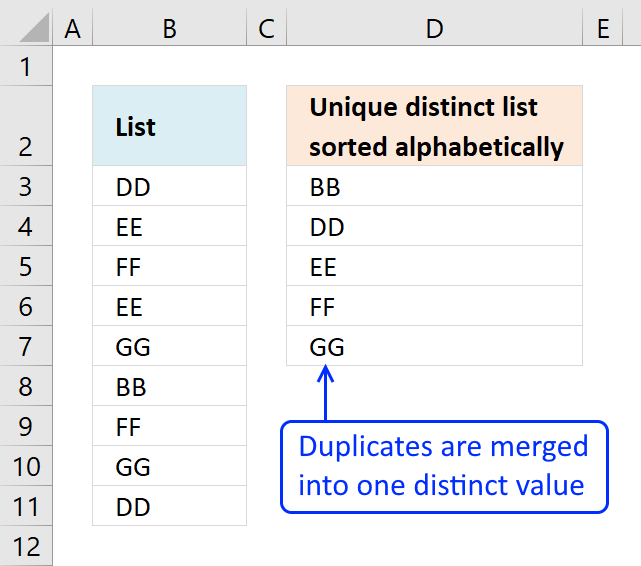Author: Oscar Cronquist Article last updated on February 11, 2018The array formula in cell D3 extracts unique distinct values sorted A to Z, from column B to column D. Unique distinct values are all values except duplicates.

Example, in column B value "DD" exists twice in cell B3 and B11. In column D value "DD" exists only once since it is a unique distinct list.

Array formula in cell D3:

=INDEX(\$B\$3:\$B\$11, MATCH(SMALL(IF(COUNTIF(\$D\$2:D2, \$B\$3:\$B\$11)=0, COUNTIF(\$B\$3:\$B\$11, "<"&\$B\$3:\$B\$11), ""), 1), COUNTIF(\$B\$3:\$B\$11, "<"&\$B\$3:\$B\$11), 0))

### Watch a video that explains how to use it and how it works:

Learn how to filter values with a condition and return unique distinct values sorted from A to Z:

Unique distinct list sorted alphabetically based on a condition

The array formula in cell E6 filters values in column C based on value in cell E3, the output is […]

### How to create an array formula

1. Double click on cell D3
2. Copy (Ctrl +c) amd paste (Ctrl+v) above formula to cell D3
3. Press and hold Ctrl + Shift simultaneously
4. Press Enter once
5. Release all keys

The formula in the formula bar should now look like this: {=formula}
Don't enter the curly brackets yourself, they appear automatically.How to enter an array formula

Array formulas allows you to do advanced calculations not possible with regular formulas.

### How to copy array formula

1. Select cell D3
2. Copy cell  (Keyboard shortcut: Ctrl + c)
3. Select cell range D4:D7
4. Paste (Keyboard shortcut: Ctrl + v)

### Explaining array formula in cell

Step 1 - Identify values not yet shown above current cell

The COUNTIF function counts the number of times a value exists in a cell range.

Cell range \$D\$2:D2 changes as the formula is copied down to cells below. This makes it possible to avoid duplicate values in the list.

COUNTIF(\$D\$2:D2,\$B\$3:\$B\$11)=0

becomes

COUNTIF("Unique list sorted alphabetically",{"DD";"EE";"FF";"EE";"GG";"BB";"FF";"GG";"DD"})=0

becomes

{0;0;0;0;0;0;0;0;0}=0

and returns

{TRUE; TRUE; TRUE; TRUE; TRUE; TRUE; TRUE; TRUE; TRUE}

Step 2 - Create an array with a ranking sort number

COUNTIF(\$B\$3:\$B\$11,"<"&\$B\$3:\$B\$11)

becomes

{1;3;5;3;7;0;5;7;1}

Step 3 - Convert array with not displayed values to an array containing rank numbers

The IF function converts not yet displayed values into alphabeically ranked numbers.

IF(COUNTIF(\$D\$2:D2,\$B\$3:\$B\$11)=0,COUNTIF(\$B\$3:\$B\$11,"<"&\$B\$3:\$B\$11),"")

becomes

IF({TRUE; TRUE; TRUE; TRUE; TRUE; TRUE; TRUE; TRUE; TRUE},{1;3;5;3;7;0;5;7;1},"")

and returns

{1;3;5;3;7;0;5;7;1}

Step 4 - Find the smallest value in array

The SMALL function extracts the smallest number in the array.

SMALL(IF(COUNTIF(\$D\$2:D2, \$B\$3:\$B\$11)=0, COUNTIF(\$B\$3:\$B\$11,"<"&\$B\$3:\$B\$11), ""),1)

becomes

SMALL({1;3;5;3;7;0;5;7;1},1)

and returns 0.

How to use the SMALL function

The SMALL function lets you extract a number in a cell range based on how small it is compared to the other numbers in the group.

Step 5 - Find relative position in the array

The MATCH function finds the position of the next alphabetically sorted value.

MATCH(SMALL(IF(COUNTIF(\$D\$2:D2, \$B\$3:\$B\$11)=0, COUNTIF(\$B\$3:\$B\$11,"<"&\$B\$3:\$B\$11),""), 1),COUNTIF(\$B\$3:\$B\$11, "<"&\$B\$3:\$B\$11), 0)

becomes

MATCH(0,COUNTIF(\$B\$3:\$B\$11, "<"&\$B\$3:\$B\$11), 0)

becomes

MATCH(0,{1;3;5;3;7;0;5;7;1}, 0)

and returns 6.

Step 6 - Return value in data based on row coordinate

The INDEX function returns a value based on row and column number.

INDEX(\$B\$3:\$B\$11, MATCH(SMALL(IF(COUNTIF(\$D\$2:D2, \$B\$3:\$B\$11)=0, COUNTIF(\$B\$3:\$B\$11, "<"&\$B\$3:\$B\$11), ""), 1), COUNTIF(\$B\$3:\$B\$11, "<"&\$B\$3:\$B\$11), 0))

becomes

INDEX(\$B\$3:\$B\$11, 6)

becomes

INDEX({"DD"; "EE"; "FF"; "EE"; "GG"; "BB"; "FF"; "GG"; "DD"}, 6)

and returns BB in cell D3.